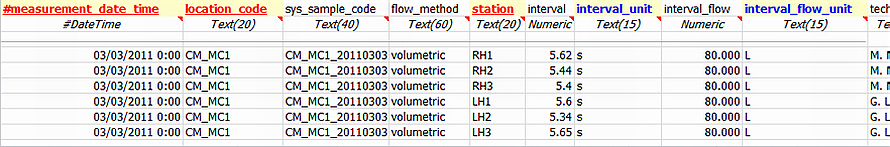﻿ Volumetric Flow Method

# Volumetric Flow Method

Navigation:  EDGE > EDGE Features > Flow Calculations >

# Volumetric Flow Method

This method calculates the instant flow (m3/sec) at a given location from field measurements based on the Volumetric Flow Method. The method requires field measurements of the time taken to fill or collect a known volume of water. The instant flow is automatically calculated as the average of all the individual flow measurements at that location, based on the following equation:

INSTANT_FLOW = INTERVAL_FLOW/INTERVAL

where:        INTERVAL_FLOW = volume filled or collected (L or m3);

INTERVAL = time taken to fill or collect the INTERVAL_FLOW volume (s)

Multiple records of instant flow taken at the same location and station will be averaged together.

1.Open the Flow_Measurement tab in EDGE.

3.Populate FLOW_METHOD with VOLUMETRIC, INTERVAL and INTERVAL_FLOW (with units). The SYS_SAMPLE_CODE should be identical for all measurements relating to a specific flow location/time.

4.Open the Flow tab. EDGE will create a new row for samples found in Flow_Measurement and populate the INSTANT_FLOW field. If more than one record for a location is found in the FLow_Measurement tab, INSTANT_FLOW will be averaged.

5.If a matching sample does not exist in the Field Samples tab, a sample will automatically created during the Create step in EDP.

Flow_Measurement Tab:Flow Tab: